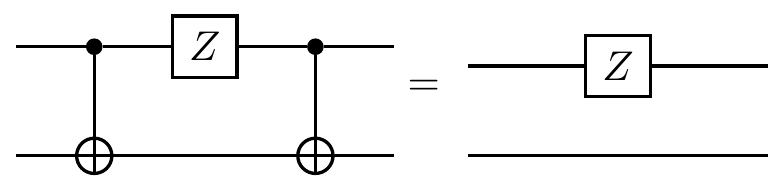## 8.5 Mathematical detour: normal subgroups

Before continuing our exploration of Pauli stabilisers, we need a bit of pure mathematics.

Let H be a subgroup of G, written H\leqslant G. We say that H is a normal subgroup of G, and write H\triangleleft G, if H is invariant under conjugation by all elements of G, i.e. ghg^{-1}\in H for all g\in G and all h\in H.

Note that we only require that ghg^{-1} be some arbitrary element in H, not that ghg^{-1}=h.

If H\leqslant G is an arbitrary (not necessarily normal) subgroup, then we can use it to “slice up” G into subsets of equal size called cosets, one of which is H itself. We define a (left) coset to be a set of the form gH = \{gh \mid h\in H\} for any fixed g\in G. Any two cosets (i.e. any two choices of g\in G) are either entirely equal or completely disjoint. The relevance of normality here is that if H is normal, then there is no need to distinguish between left (gH) and right (Hg) cosets, and in this case we can construct the quotient group G/H consisting of cosets with the operation defined by gH\cdot g'H=(gg')H. Here is one way to visualise a partition into cosets:The bottom row represents the subgroup H, and each row above represents a coset, i.e. a set of elements generated by picking an element c_k of G that does not belong to H nor to any of the previously generated cosets, and then multiplying this element by all elements in H, one at a time. This picture above shows that, for any finite group G and any subgroup H\leqslant G, |G| = |G:H|\cdot|H| where |G:H| is the number of cosets of G given by H. This fact is known as Lagrange’s theorem (although Joseph-Louis Lagrange was a rather prolific mathematician, working in many areas, so this is only one of the theorems to bear his name).

It seems like it was Évariste Galois who recognised that normal subgroups were worthy of special attention. Given an arbitrary subgroup H\leqslant G, we can construct a larger subgroup K\leqslant G in which H is normal, i.e. such that H\triangleleft K\leqslant G. The largest such subgroup K is called the normaliser of H in G, denoted by N_G(H), and we can construct it explicitly: N_G(H) = \{g\in G \mid ghg^{-1}\in H\text{ for all }h\in H\}. In words, the normaliser consists of the set of elements of G that conjugate all elements of H to elements of H. This suggests a very subtle question: is every subgroup of a normal subgroup normal? The answer is most definitely no: if H\triangleleft K and K\triangleleft G then it is not necessarily the case that H\triangleleft G, merely that H\leqslant G.

As we shall soon see, Pauli stabilisers are not normal subgroups of \mathcal{P}_n, and we will instead want to study their normalisers.